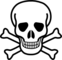# a deSolve pitfall

Beware!As suggested in the ode() examples, I typically use a with() statement in my gradient function for convenience, so I don't have to refer to the order of the parameters in the parameter vector or the order of the variables in the state vector. I thought this meant that I didn't have to worry about ordering at all, but that's not true. Here's an example/explanation.

Set up a simple linear model ($$x$$ exponentially increasing, $$y$$ exponentially decreasing):

library("deSolve")
gradfun <- function(t, statevec, params) {
g <- with(as.list(c(statevec, params)), c(x = a * x, y = b * y))
list(g)
}
params0 <- c(a = log(2), b = -log(2))
tvec <- 0:5


Starting at x=0, y=1, the right way:

ode(c(x = 0, y = 1), tvec, gradfun, params0)

##   time x       y
## 1    0 0 1.00000
## 2    1 0 0.50000
## 3    2 0 0.25000
## 4    3 0 0.12500
## 5    4 0 0.06250
## 6    5 0 0.03125


As should happen, $$x$$ remains at zero and $$y$$ decreases exponentially back toward zero.

What happens if we get the order of the initial conditions wrong (even though the names are right?)

ode(c(y = 1, x = 0), tvec, gradfun, params0)

##   time       y       x
## 1    0  1.0000  0.0000
## 2    1  0.7692 -0.6390
## 3    2  0.1835 -0.9830
## 4    3 -0.4870 -0.8734
## 5    4 -0.9327 -0.3607
## 6    5 -0.9479  0.3185


The system is now cross-wired, equivalent to

$\begin{split} \dot x & = -y \\ \dot y & = x \end{split}$

cbind(cos(log(2) * tvec), -sin(log(2) * tvec))

##         [,1]    [,2]
## [1,]  1.0000  0.0000
## [2,]  0.7692 -0.6390
## [3,]  0.1835 -0.9830
## [4,] -0.4870 -0.8734
## [5,] -0.9327 -0.3607
## [6,] -0.9479  0.3185


What is happening here?

• The gradient gets computed according to the names of the vector; because of the way we set the initial values vector, $$y$$ is the first element and $$x$$ is the second element.
• all other internal stuff deals with the vectors by position (i.e. ignoring names), so the first computed gradient, which is supposed to be applied to the $$x$$ component, gets applied to the first element (which is the $$y$$ component) instead, so we end up with the cross-wired solution.

## Hacked solution

This is a workable solution, I think: it depends on the user having assigned appropriate names to the gradient vector (this is good practice in any case).

myCheckFunc <- deparse(deSolve:::checkFunc)
myCheckFunc <- c(myCheckFunc[1:3], "   if (!all(names(tmp[])==names(y))) {",
"warning('name mismatch between y and results of grad()')", " }", myCheckFunc[4:length(myCheckFunc)])
myCheckFunc <- eval(parse(text = myCheckFunc), envir = environment(deSolve:::checkFunc))
assignInNamespace("checkFunc", myCheckFunc, ns = "deSolve")

ode(c(y = 1, x = 0), tvec, gradfun, params0)

## Warning: name mismatch between y and results of grad()

##   time       y       x
## 1    0  1.0000  0.0000
## 2    1  0.7692 -0.6390
## 3    2  0.1835 -0.9830
## 4    3 -0.4870 -0.8734
## 5    4 -0.9327 -0.3607
## 6    5 -0.9479  0.3185


No warning if the gradient vector is not named (it would be possible to add this check, i.e. warn if y has names but the result of grad() doesn't).

gradfun2 <- function(t, statevec, params) {
g <- with(as.list(c(statevec, params)), c(a * x, b * y))
list(g)
}
ode(c(y = 1, x = 0), tvec, gradfun2, params0)

##   time       y       x
## 1    0  1.0000  0.0000
## 2    1  0.7692 -0.6390
## 3    2  0.1835 -0.9830
## 4    3 -0.4870 -0.8734
## 5    4 -0.9327 -0.3607
## 6    5 -0.9479  0.3185